# Fair dividend rate foreign currency hedges

(Clauses 9, 24, 33 and 57)

### Summary of proposed amendment

At present, there is a mismatch in the tax treatment of foreign currency hedges and certain offshore assets – those that are taxed under the fair dividend rate (FDR) rules and certain Australian Stock Exchange (ASX) listed shares. This mismatch makes it more difficult to effectively hedge investments in certain offshore assets.

To address this, the bill provides for a new, optional rule that effectively allows eligible taxpayers to apply FDR to their foreign currency hedges rather than the financial arrangement rules. This new rule is designed to, as much as possible, eliminate the tax mismatch.

To ensure this new rule is robust, there will be restrictions on when the new rule can be applied to ensure that it is used as intended.

### Application date

The new rule will come into effect from the beginning of the 2013–14 income year.

### Key features

• The bill will create new subpart EM, which provides for a new tax calculation method for certain foreign currency hedges entered into for assets taxed under FDR or ASX-listed shares that are not subject to the foreign investment fund (FIF) rules provided the sale of those shares would not be taxable.
• The new rule will be optional. Eligible taxpayers will be able to elect what hedges it will apply to, and to what extent it will apply for each hedge (subject to maximums to ensure the use of the new rule is appropriate).
• The new rule will apply only to widely held entities to help ensure it is used only as intended. Such entities generally have muted incentives to take aggressive tax positions, have investment mandates and other documentation that disclose investment strategies.

### Background

At present, there is a mismatch in the tax treatment of foreign currency hedges and certain offshore assets. For example, under the FDR rules, changes in an asset’s value are not taxed. Instead, FDR assets are generally taxed on an imputed return of 5 percent. Conversely, changes in a hedge’s value are fully taxed under the financial arrangement (FA) rules. This mismatch in treatment means that a hedge that is effective in removing the impact of unexpected currency fluctuations before tax ceases to be effective after tax.

To illustrate, say a person has an offshore asset portfolio worth US\$10,000 and the NZD/USD exchange rate unexpectedly rises from \$0.75 to \$0.80. The person’s asset portfolio is taxed under the FDR rules. In New Zealand dollars, the portfolio’s value falls from NZ\$13,333.33 to NZ\$12,500.00. If the person had used a foreign currency hedge to completely remove exchange rate risk, before tax is taken into account, the hedge will increase in value by NZ\$833.33, exactly cancelling the change in their portfolio’s value. The hedge is totally effective before-tax.

The story is different after-tax. The offshore assets have lost NZ\$833.33 of value. However, under the FDR rules, no deduction is given for this decrease. Despite this, the \$833.33 increase in the hedge’s value is taxable. After-tax, the person has lost NZ\$833.33 from their asset portfolio but gained only NZ\$600.00 from their hedge; the shortfall of \$233.33 is created by the tax payment.

Concept of a hedge

In essence, a basic foreign currency hedge is a currency swap. At some future date (the “contract date”), a person promises to exchange a fixed amount of foreign currency (“foreign amount hedged”) with a fixed amount of domestic currency (“domestic amount hedged”). Because the amount of foreign and domestic currency is fixed when the swap is entered into, this has the effect of “locking in” a future exchange rate for the person.

The value of a foreign currency hedge is measured as the difference between the hedged exchange rate and the market’s best guess of what the exchange rate will be on the contract date. The fair value of a hedge should begin at zero as the “locked in” exchange rate will equal the market’s best guess of what the exchange rate will be on the contract date.

In later periods the forward rate for the contract date may change. If this happens it will drive a wedge between the contract rate and the forward rate, increasing or decreasing the value of the hedge.

For example, say a hedge allows an individual to exchange (sell) US\$100 (i.e. the foreign amount hedged) for NZ\$200 (ie the domestic amount hedged) (contract rate of 0.50) for value 1 January 2011 (the contract date). This contract rate will be the same as the market calculated forward rate of 0.50 for 1 January 2011. The value of the hedge contract is therefore \$0 – the contract and forward rates are the same.

If at a later date the forward rate for value 1 January 2011 moves to \$0.60, the unrealised value of the hedge will increase to \$33.33. The reason for this is because the change in the forward rate indicates that it is now expected for the exchange rate on 1 January 2011 will be \$0.60. Accordingly, when the hedge is realised the individual would expect to make \$33.33 (they will give up US\$100, which at the current exchange rate is worth NZ\$166.66, and in return will receive NZ\$200).

Importantly, in order for something to be a “hedge” it must be used to back an asset denominated in a foreign currency. This means that changes in the value of the hedge will offset changes in the foreign denominated asset. The foreign amount hedged in the currency being hedged cannot exceed the value of assets denominated in that currency.

To illustrate, say in the example above the individual had US\$100 of USD-denominated assets. Because of the change in the exchange rate from \$0.50 to \$0.60, the value of these assets in NZD would fall from NZ\$200 to NZ\$166.66. However, the hedge has increased in value by NZ\$33.33, cancelling out this fall. If, however, the individual had no USD-denominated assets, they would have suffered no loss but have made a gain on the foreign currency swap. Thus the swap is not a true “hedge” – it is just currency speculation. Such speculation should rightly be taxed under the financial arrangement rules.

The apportionment rules and quarterly test described below are designed to ensure the new rule covers only true hedges.

### Detailed analysis

#### New calculation for income or deductions from a hedge

New section EM 6 is the core of the new rule. It will provide that a taxpayer has income or an expense of: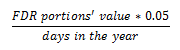FDR portions’ value is the current market value of a taxpayer’s hedges to the extent that the taxpayer has elected for this rule to apply to those hedges. This calculation will need to be carried out every day. Because hedges are generally short term, this calculation must be performed numerous times over a hedge’s life for it to be effective.

This daily calculation aligns with how eligible entities calculate their tax liability on eligible assets. The tax calculation for FDR assets is based on an imputed 5 percent return and is done on a daily basis – a calculation that is very similar to the above. The calculation also closely matches the tax treatment of ASX-listed shares that are not subject to the FIF rules (meaning tax is simply levied on dividends received) as the estimated dividend yield from such a share is 5 percent per year.

The bill also inserts new sections CV 18 and DV 25, which will provide that the income or expense calculated using the above formula is taxable or deductible (as applicable). Note that section DV 25 does not override the general permission, so any deemed expense that arises under section EM 6 will need to be related to a taxpayer’s business in order for it to be deductible. The capital limitation will be overridden to ensure that a negative result of the above formula arising due to eligible ASX-listed shares held on capital account is deductible.

#### Financial arrangement rules will not apply

New section EM 1(3) provides that the financial arrangement rules do not apply to a hedge to the extent a taxpayer has elected for this new rule to apply under section EM 4 (its fair dividend rate hedge portion). Tax on the hedge will be calculated solely under subpart EM.

The financial arrangement rules will apply as normal to the remainder of the hedge.

#### Eligible assets

The new tax calculation method will be available in relation to hedges that hedge:

• assets that are taxed under the FDR method; and
• shares listed on the ASX exchange which are not subject to the FIF rules provided the sale of those shares would be exempt under section CX 55 or would be a capital receipt as the shares are held on capital account.

The purpose of the new rule is to align the tax treatment of foreign currency hedges with the assets those hedges are entered into for. This mismatch is most significant for hedges entered into for the asset types listed above. For other assets the mismatch is either much less pronounced or does not exist, so the current treatment is more appropriate.

In addition to this asset-type requirement, an asset’s market value must also be calculated on a daily basis in order for it to be an eligible asset. This is so the calculation in section EM 6 can be carried out daily, as set out above.

#### Eligible entities

The new rule is designed to apply only to widely held investment funds and other similar entities. This restriction is designed to help ensure the new rule will be used only as intended. Widely held funds generally have muted incentives to take aggressive tax positions and have investment mandates and other documentation that disclose investment strategies.

This restriction will be provided by new section EM 2, which will largely mimic the widely held entity criteria in the Portfolio Investment Entity (PIE) rules (sections HM 14 and HM 15, together with the exemptions in sections HM 21 and HM 22).

#### Eligible hedges and hedge portions

The new rule can only be used in relation to genuine foreign currency hedges – financial arrangements that are entered into with the sole purpose of offsetting exposure to foreign currency exchange movements in the value of their assets.

To reflect this, new section EM 3 provides the criteria for an eligible hedge. For example, a hedge must not be entered into with an associated person and, when it is first entered into, must have a fair value of zero.

An eligible hedge will not automatically be subject to the new tax calculation. A taxpayer will need to elect, on the day it enters into a hedge, that the new tax calculation is to apply.

The new calculation will be able to be applied to part or all of a hedge. Accordingly, the taxpayer’s election will need to include the portion of the hedge to be subject to the new calculation (the fair dividend rate hedge portion of the hedge), although there are limits on this (see below).

There will be no prescribed way for how this election is to be made, but sufficient record of elections must be kept in order to satisfy the general record-keeping requirements placed on taxpayers.

#### Maximum fair dividend rate hedge portions

The intention of the new rule is that it should only apply to hedges entered into for certain types of offshore investment – those assets where the tax mismatch is most significant. It is appropriate that hedges entered into for other types of offshore investment continue to be taxed as they are currently. Accordingly, new section EM 5 provides rules that set out the maximum fair dividend rate hedge portion that a taxpayer can elect.

There are two possible methods that a taxpayer will be able to use to determine this maximum. A taxpayer will be required to use the same calculation method for all of its hedges. The first method is designed to be accurate but may be relatively complicated to apply. The second method is simpler but may be less accurate so, to balance this, it is more restrictive.

These calculations will only need to be performed on the day a hedge is first entered into. There will be a quarterly test, described below, to ensure that a hedge’s initial fair dividend rate hedge portion remains appropriate over time.

##### Method one

The first calculation method will use the formula: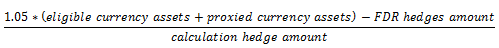Eligible currency assets will be eligible assets (as described above) denominated in the same currency as the hedge being entered into (the calculation currency). Proxied currency assets will be eligible assets denominated in a different currency but the taxpayer hedges that currency with the calculation currency. All amounts in the formula will be expressed in the calculation currency.

FDR hedges amount will be the amount of the calculation currency that is hedged by the taxpayer’s hedges, excluding the hedge that the calculation is being carried out for (the calculation hedge), to the extent that they have elected for this new tax calculation method to apply (i.e. each hedge’s fair dividend rate hedge portion).

Calculation hedge amount is the amount of foreign currency that is hedged by the calculation hedge.

Example

Z has a portfolio of:

• US\$20,000 worth of shares in US-based companies (eligible assets, worth NZ\$40,000)
• AU\$10,000 worth of shares in Australian companies (eligible assets, worth NZ\$15,000)
• AU\$20,000 worth of Australian bonds (non-eligible assets, worth NZ\$30,000)

Z has currently has two foreign currency hedges:

• A hedge for US dollars with a foreign amount hedged of \$20,000 (equivalent to NZ\$40,000) and a fair dividend rate hedge portion of 0.50.
• A hedge for Australian dollars with a foreign amount hedged of \$20,000 (equivalent to NZ\$30,000) and a fair dividend rate hedge portion of 0.25.

Z is looking to enter into a new hedge for AU\$10,000. The maximum fair dividend rate portion for the hedge would be 0.55: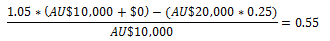##### Method two

The second calculation method uses the formula: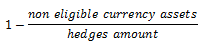Unlike method one, this calculation will be made with reference to a taxpayer’s assets regardless of the currency they are denominated in, not just the calculation currency. This may make it simpler for some taxpayers.

Non eligible currency assets will be the total value of a taxpayer’s foreign assets excluding eligible assets, converted to New Zealand dollars. Hedges amount will be the total amount of foreign currency that is hedged by a taxpayer’s hedges including the calculation hedge, again converted to New Zealand dollars. Importantly, this is not the amount of New Zealand dollars hedged; it is the amount of foreign currency hedged expressed in New Zealand dollars at the day’s prevailing exchange rate.

To ensure a taxpayer cannot over-hedge using method two, if the result of the formula below is above 1.05, a taxpayer will not be able to elect to use the new method for new hedges.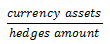Currency hedges will be the total value of the taxpayer’s offshore assets converted to New Zealand dollars. Hedges amount will be the same as in the previous formula.

Example

Z from the example above decides instead to use the second method to calculate the maximum fair dividend rate portion for its new \$10,000 Australian dollar hedge. This hedge is equivalent to NZ\$15,000.

The value of Z’s non-eligible assets in New Zealand dollars is \$30,000. The total amount of foreign currency hedged by Z, expressed in New Zealand dollars, is \$85,000. Accordingly, the hedge’s maximum fair dividend rate hedge portion will be 0.65 unless the result of second formula below is greater than 1.05:

First formula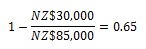Second formula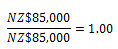The second formula’s result is 1.00, so the maximum fair dividend rate hedge portion of 0.65 stands.

#### Quarterly test

The two proposed apportionment methods described above will provide the appropriate maximum fair dividend rate hedge portions initially. However, a quarterly test is also required to ensure the taxpayer’s initial allocation is still appropriate.

The formula below provides a taxpayer’s quarterly FDR hedging ratio: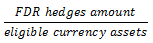FDR hedges amount will be the total amount of foreign currency that is hedged by a taxpayer’s hedges to the extent the taxpayer has elected for this tax calculation to apply to those hedges (i.e. the fair dividend rate hedge portion of each hedge), converted to New Zealand dollars. Again this will not be the amount of New Zealand dollars hedged but is the amount of foreign currency hedged expressed in New Zealand dollars at the day’s prevailing exchange rate. Eligible currency assets will be the total value of the taxpayer’s eligible assets, also converted to New Zealand dollars.

If a person’s quarterly FDR hedging ratio is greater than 1.05 they must adjust the fair dividend rate hedge portion to the result of the formula below: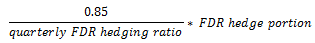The effect of this formula will be to bring an entity’s quarterly FDR hedging ratio to 0.85. This adjustment will be required to be carried out, at the most, five days after the end of the quarter.

If a taxpayer breaches the threshold in two consecutive quarters, they will not be able to use this new tax calculation method for the remainder of the income year and the next income year for any new hedges it enters into.

Example

At the end of a quarter, the value of Y’s portfolio is:

• US\$10,000 worth of shares in US-based companies (eligible assets, worth NZ\$20,000)
• AU\$20,000 worth of shares in Australian companies (eligible assets, worth NZ\$30,000)

Y currently has a single foreign currency hedge: a hedge for US dollars with a foreign amount hedged of \$20,000 (equivalent to NZ\$40,000) and a fair dividend rate hedge portion of 0.40.

In the first formula, FDR hedges amount is NZ\$16,000 (=\$40,000 * 0.40) and eligible currency assets is NZ\$50,000 (=\$20,000 + \$30,000). The result of the formula is 0.32: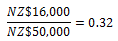Y will therefore pass the quarterly test.

Say next quarter Y enters into a hedge in addition to its USD hedge: a hedge for AU\$30,000 (equivalent to NZ\$45,000) with a fair dividend rate hedge portion of 1.00 (note: this would exceed the maximum fair dividend rate hedge portion allowed, but for simplicity is ignored).

If the exchange rate or prices do not change, FDR hedges amount will now be NZ\$61,000 (=\$40,000 * 0.40 + \$45,000 * 1.00) but eligible currency assets remains at NZ\$50,000. The result of the formula is now 1.22: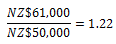This exceeds 1.05, so Y will have to adjust its two hedges as follows so the fair dividend rate hedge portions on its US dollar and Australian dollar hedges are 0.28 and 0.70, respectively.

US dollar hedge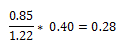Australian dollar hedge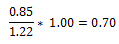3 It is generally impractical to hedge every currency a fund is exposed to. Funds therefore often do what is referred to as “proxy hedging”. They find correlations between the smaller currencies and larger ones (such as the USD), and hedge their exposure to the smaller currencies using the larger ones.